Contents Articles Behaviors Books Director News Director Web Sites FAQ Games Mailing Lists News Groups Project Examples Reviews Software Tools Useful Web Sites Utilities Xtras

 Don't miss these#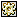Useful string functions

 Compatibilities: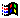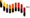Rating: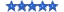Author: LukeWigley

Functions for searching and manipulating strings

 -- Functions - Manipulating Strings on findWordfromChar str, charPos   -- returns the word that the specified character occurs in   wordBreak = [SPACE, TAB, ",", ".", ":", ";", "?", "!", RETURN]   if getOne(wordBreak, str.char[charPos]) > 0 then return EMPTY   mx = length (str)   fWrd = str.char[charPos]   i = charPos - 1   repeat while i > 0     if getOne(wordBreak, str.char[i]) > 0 then       exit repeat     else       fWrd = str.char[i] & fWrd       i = i -1     end if   end repeat   i = charPos + 1   repeat while i <= mx     if getOne(wordBreak, str.char[i]) > 0 then       exit repeat     else       fWrd = fWrd & str.char[i]       i = i + 1     end if   end repeat   return fWrd end on getRandomChar letCase   -- returns a random letter; if case is not specifed (as #upper or #lower), it picks a random case   if not symbolP(letCase) then set letCase = getAt([#upper, #lower], random(2))   if letCase = #upper then set aLet = 64 + random(26)   else set aLet = 93 + random (26)   set lett = numToChar (aLet)   return lett end on capitalise lett   -- if the param is a lower case letter, an uppercase version is returned   if stringP(lett) then     set tA = charToNum(lett)     if tA = min(max(96, tA), 123) then       set tA = tA - 32         set lett = numTOChar (tA)     end if   end if   return lett end on forceSentenceCase str   -- converts the first letter of the first word of the str to uppercase --  str = forceLowerCase (Str)   set lett = char 1 of str   set tA = charToNum(lett)   if tA = min(max(96, tA), 123) then     set tA = tA - 32       set lett = numTOChar (tA)   end if   set outPut = lett & char 2 to length(str) of str   return output end on CapitaliseFirstLetter str   -- converts the first letter of each word to uppercase   set wCount = the number of words in str   set outPut = ""   repeat with j = 1 to wCount     set wrd = word j of str     set lett = char 1 of wrd     set tA = charToNum(lett)     if tA = min(max(96, tA), 123) then       set tA = tA - 32         set lett = numTOChar (tA)     end if     put lett & (char 2 to length (wrd) of wrd) & " " after output   end repeat   delete the last char of output   return output end on forceUppercase iStr   -- takes a string and converts it to all uppercase   set oStr = ""   set n = length(iStr)   repeat with i = 1 to n     set tA = charToNum(char i of iStr)     if tA = min(max(97, tA), 122) then set tA = tA - 32   -- char is lowercase     put numTOChar (tA) after oStr   end repeat   return oStr end on forceLowercase iStr   -- takes a string and converts it to all lowercase   set oStr = ""   set n = length(iStr)   repeat with i = 1 to n     set tA = charToNum(char i of iStr)     if tA = min(max(65, tA), 90) then set tA = tA + 32       put numTOChar (tA) after oStr   end repeat   return oStr end on forceTitleCase iStr   set oStr = ""   set lStr = forceLowerCase (iStr)   set mWrd = the number of words in lStr   repeat with j = 1 to mWrd     set wrd = word j of lStr     set fChar = char 1 of wrd     set UChar = forceUppercase (fChar)     delete char 1 of wrd     put Uchar & wrd & " " after oStr   end repeat   delete the last char of oStr   return oStr end on searchAndReplace input, oldStr, newStr   -- searches the input (string) for oldStr and replaces it with newStr   set output = ""   repeat while input contains oldStr     set posn = offset(oldStr, input)-1     if posn > 0 then put char 1 to posn of input after output     put newStr after output     delete char 1 to (posn + length(oldStr)) of input   end repeat   put input after output   return output end on trimPunctuation pStr   set str = pStr   set punc = " ;:,.-?!*"   repeat while (length (str) > 0) AND (punc contains char 1 of str)     delete char 1 of str   end repeat      repeat while (length (str) > 0) AND (punc contains the last char of str)     delete the last char of str   end repeat      return str end on trimChars charsToTrim, pStr   set str = pStr   repeat while (length (str) > 0) AND (charsToTrim contains char 1 of str)     delete char 1 of str   end repeat      repeat while (length (str) > 0) AND (charsToTrim contains the last char of str)     delete the last char of str   end repeat      return str end on removeLeadingSpaces pStr   repeat while char 1 of pStr = " "     delete char 1 of pStr   end repeat   return pStr end on removeTrailingSpaces pStr   repeat while (the last char of pStr = " ")     delete the last char of pStr   end repeat   return pStr end on stripNonAlphabetChars istr   -- takes a string and strips out non-aphabetcharacters (including spaces)   set oStr = ""   set n = length(iStr)   repeat with i = 1 to n     set tA = charToNum(char i of iStr)     if (tA = min(max(96, tA), 123)) OR (tA = min(max(63, tA), 91)) then       put numToChar (tA) after oStr     end if   end repeat   return oStr end on trimSpaces pStr   set pStr = string (pStr)   -- remove leading spaces   repeat while char 1 of pStr = " "     delete char 1 of pStr   end repeat   -- remove trailing spaces   repeat while (the last char of pStr = " ")     delete the last char of pStr   end repeat   return pStr end on getASCIgarbage strLength   -- generates a string of garbage   if Not integerP(strLength) then set strLength = 100   set str = ""   repeat with k = 1 to strLength     put numToChar (random(231)) after str   end repeat   return str end on pad sourceStr, minLength, padChar, frontorBack   set str = sourceStr   if frontorBack = #front then     repeat while (length(str) < minLength)       set str = padChar & str     end repeat   else     repeat while (length(str) < minLength)       put padChar after str     end repeat   end if   return str end on stringContainsVowels str   -- returns 0 if the str contains a vowel   set vowels = ["a", "e", "i", "o", "u"]   repeat with v in vowels     if str contains v then return 0   end repeat   return -1 end on sortField fName   -- sorts field alphanumerically, line by line   set mx = the number of lines in field fName   set tList = []   repeat with j = 1 to mx     append tList, line j of field fName   end repeat   sort tList   set str = EMPTY   repeat with j = 1 to mx     put getAt (tList, j) & return after str   end repeat   delete the last char of str   put str into field fName end on stripEmptyLines str   rStr = EMPTY   mx = the number of lines in str   repeat with L = 1 to mx     chnk = line L of str     if the number of chars in chnk> 0 then put chnk & return after rStr   end repeat   delete the last char of rStr   return rStr end

 Contact MMI 36 South Court Sq Suite 300 Newnan, GA 30263 USA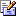Send e-mail